# Lines

## Lines - Definition, Types and Equation

Everything in the world right from a small flower to an area in the map is made up of a set of dots put together in various forms.

The most basic and elementary form of geometry is the line.

It is a one-dimensional figure having no thickness and extending infinitely from the extreme ends. It is also known as a straight line, or a right to emphasize the fact that it does not have any curves anywhere along its length. They have the ability to be embedded in higher dimensional spaces.

In Ancient time, Mathematicians introduced the concept of a line with the sole purpose of denoting objects which had no curves or were straight with negligible depth and breadth. Famous mathematician defined the line as "breadthless length".A line has a start and an end point. Let's say the points are A and B.

We represent a line by placing two arrows at both endpoints pointing in opposite directions. It symbolizes the fact that the line is extending in both directions.

TYPES OF LINES

There are various types of lines. Let us discuss the formulae, figures, and definitions of each one of it.

There are five types of lines, they are:

1.Vertical Line
2.Horizontal line
3.Parallel line
4.Skew line
5. Perpendicular lines

VERTICAL LINE

Vertical Line is a straight line, or in layman’s terms, a standing line. These type lines have an undefined slope.

We can draw a graph for a vertical line by plotting x = n. Here, n is equivalent to any sort of real number. All points in the vertical line have the same x coordinate. It is parallel to the y-axis of the coordinate plane.

The equation of the vertical line is, x = a. Here x is any point in the line of coordinate x, and a is the x-intercept.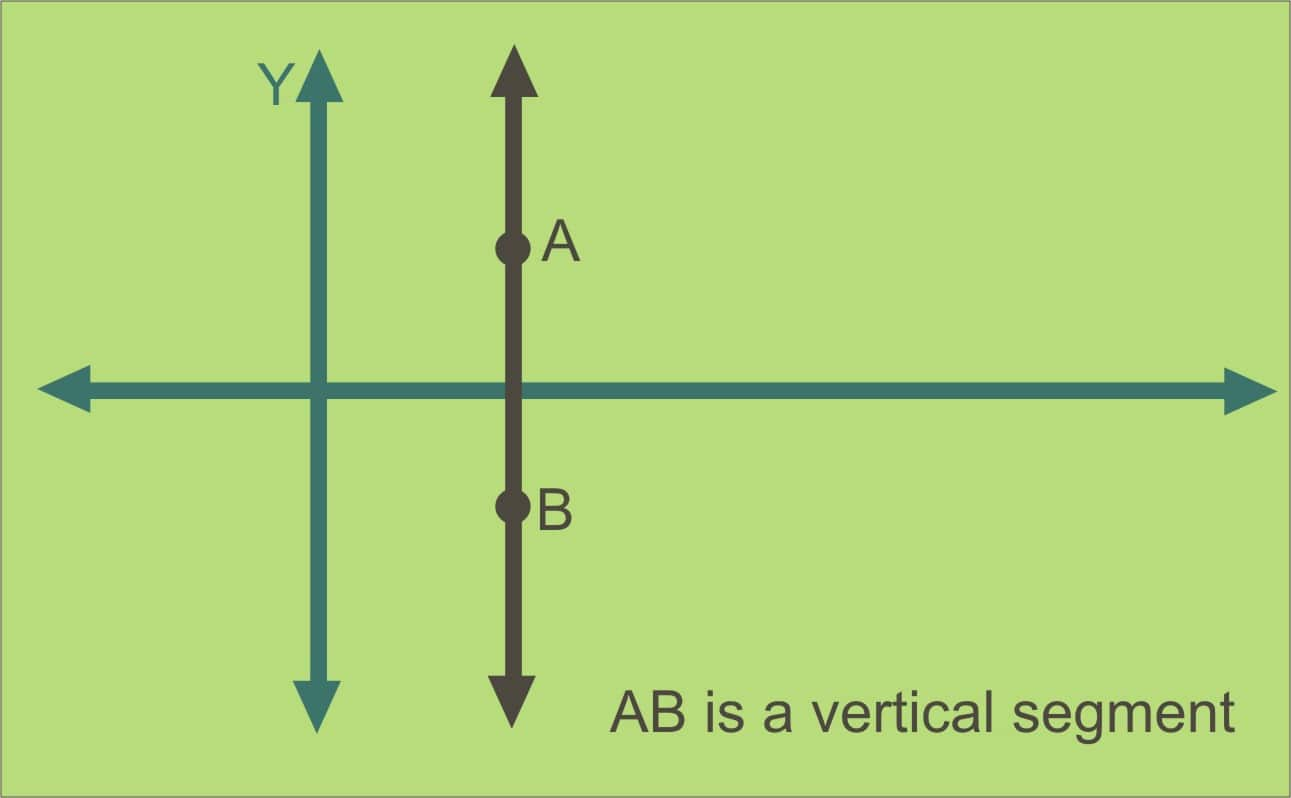HORIZONTAL LINE

Horizontal Line is one of the types of lines in which all points have the same y - coordinate. It is also called sleeping line. It is parallel to the x-axis of the plane. The slope of the horizontal line is zero.

The horizontal line equation is y = b. Here y is any point in the line of x coordinates, and b is the y-intercept. It is independent on x.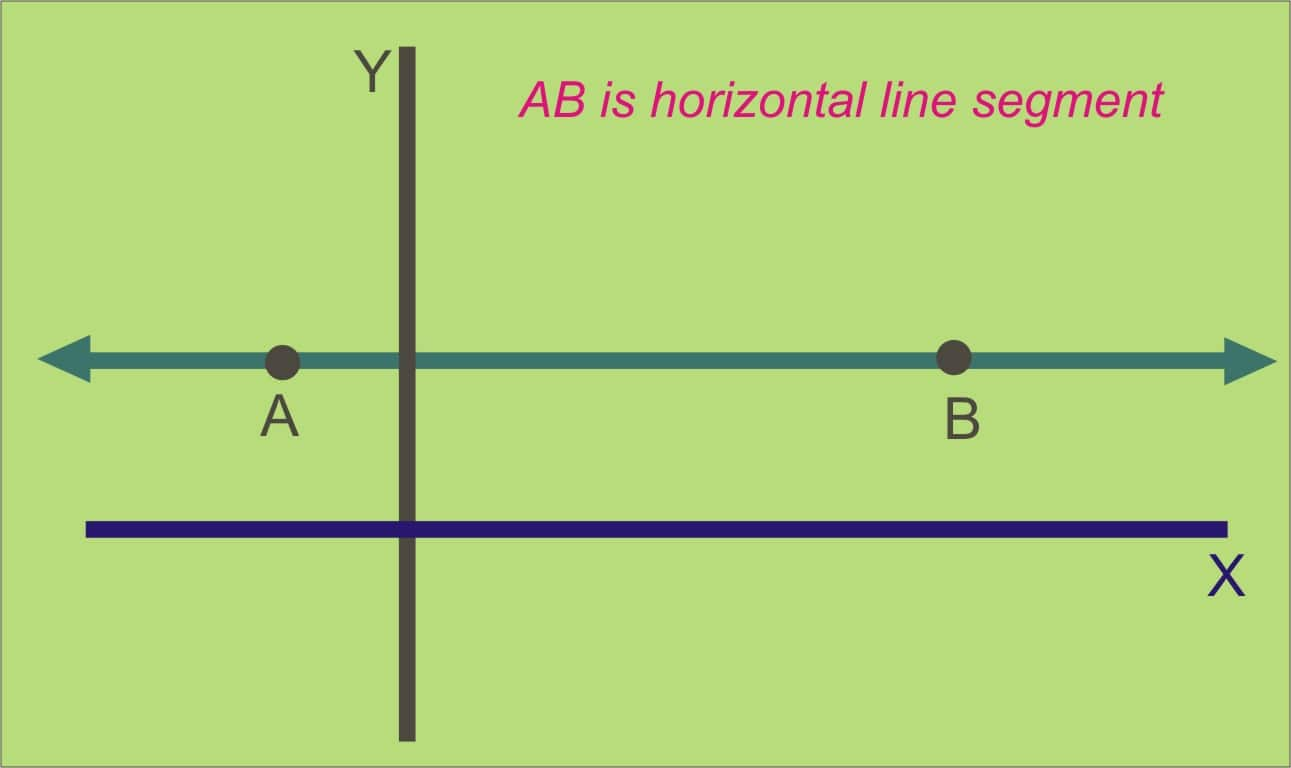PARALLEL LINE

Two lines are said to be parallel when the distance between the two straight lines is the same at all points. These two lines do not intercept at any point in space. They never meet at a point.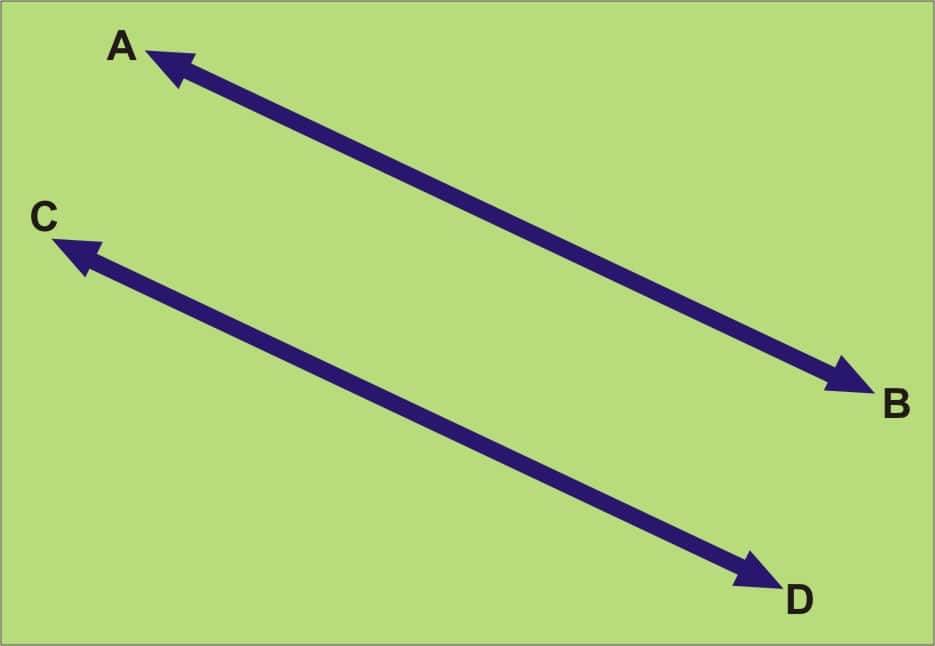PERPENDICULAR LINE

Perpendicular lines are formed when a horizontal and a vertical line meet each other at a point. They are two lines that form a congruent adjacent angle to each other. The angle formed between the vertical and the horizontal line is ninety degrees.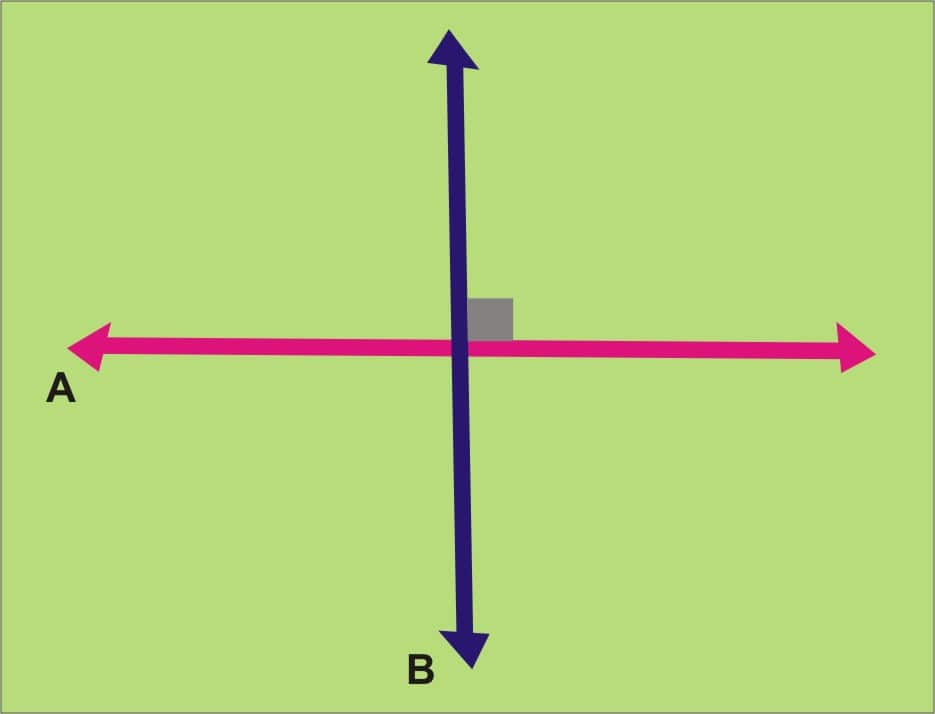Some Basic Definitions

Line segment: A line segment has two endpoints with a fixed length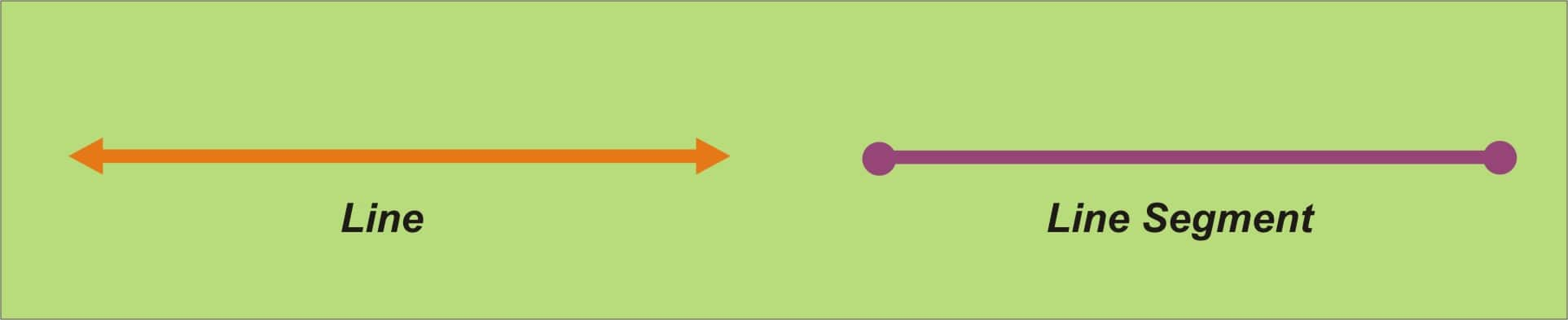Ray: A ray has one endpoint, and the other end infinitely extends in one direction.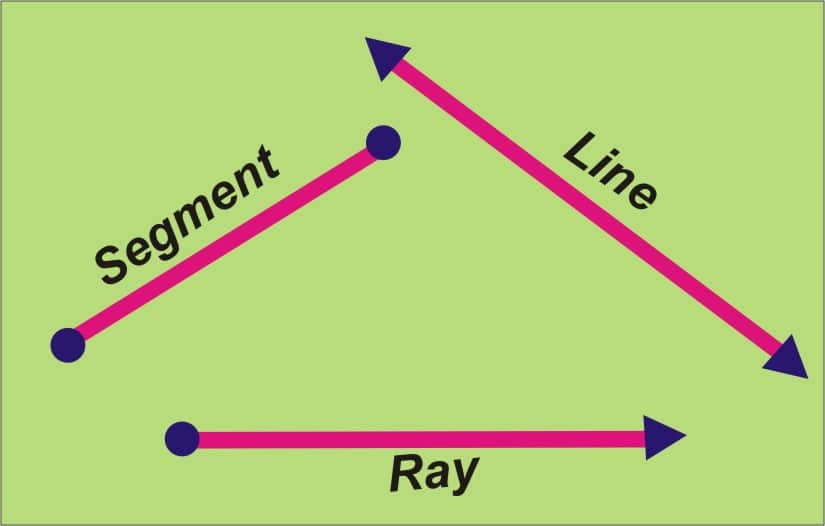Straight line: A straight line has neither starting nor endpoint and is of infinite length.
THE EQUATION OF A LINE

The standard form of line equation is Ax + By = C where A, B, and C are real numbers, A > 0 and x, y are variables. The standard form of line equation can also be written as Ax + By - C = 0.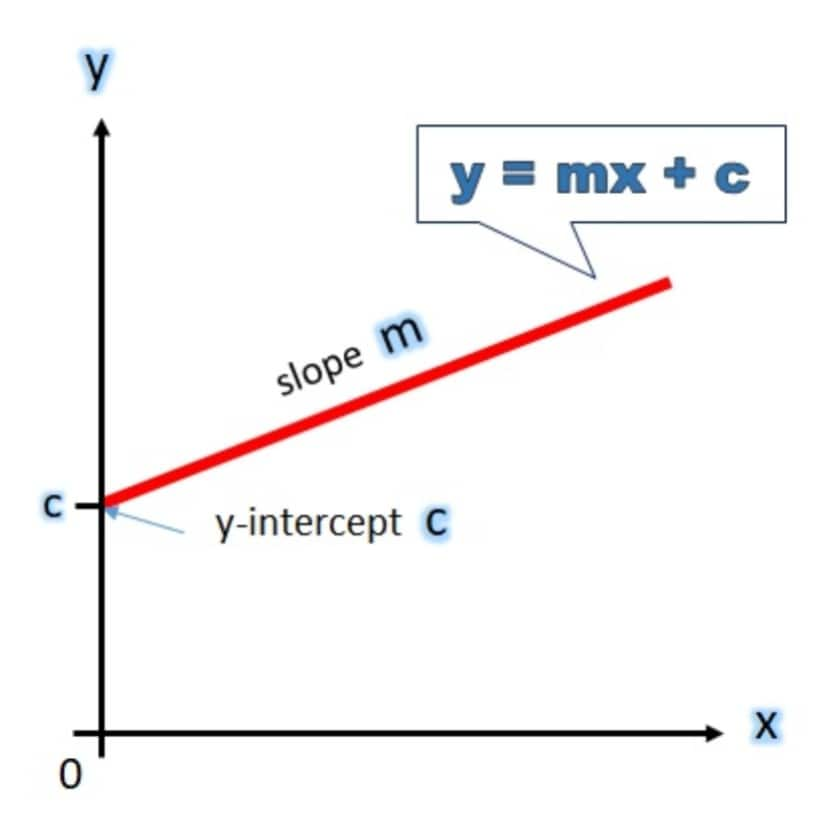Standard Form of Equation of a Line

The standard form of a line is in the form Ax + By = C, where A, B, and C are integers, it gives all of the same information as the slope-intercept form, y = mx + c.
The equation of a straight line parallel to the x-axis
The line y = k is parallel to the x-axis lies above or below the x-axis according to k which is positive or negative.
The equation of the straight line parallel to the x-axis is independent of x.
The equation of a straight line parallel to the y-axis
The line x = k is parallel to y-axis lies right or left of the y-axis according to as k is positive or negative. The equation of the straight line that is parallel to the y-axis is independent of y.
The Slope-intercept form
The equation of a straight line is y = mx + c. It has a slope m and makes an intercept c on the y-axis.
The Point-Slope Equation of a Line
The equation of a straight line passing through a fixed point and having a given slope m is called a point-slope form.
y−y1=m(x−x1), is the general equation of the straight line passing through the fixed point (x1,y1) and having slope m.
The equation of Line from Two Points
The equation of a straight line going through two fixed points also called a two-point form.
y−y1=m(x−x1), is the general equation of a straight line passing through the fixed points (x1,y1), (x2,y2) and having slope m.
where, m = y2−y1/x2−x1.